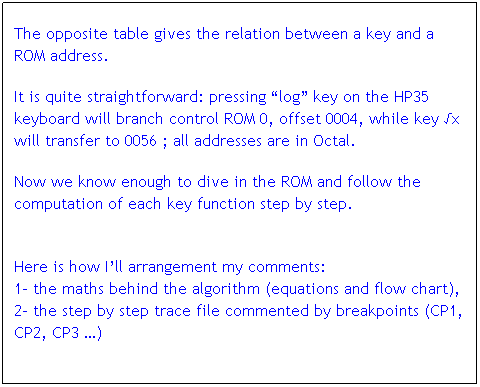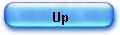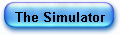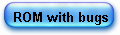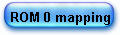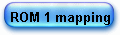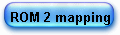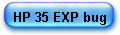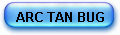HP 35 ROM step by step Announced as "a fast, extremely accurate electronic slide rule", the machine marked a milestone in computer history as being one of the first, user-friendly, micro-calculator. I reopened recently my 1970-1980 note books and thought the notes taken could be of interest for younger engineers, as I lived the micro computing revolution as an active witness. Obviously, information given in this series of articles does not infringe any copyright. Most of them are taken directly in the text of publicized (and expired) US patents or deduced by observations and measures made directly on my own old machine (yes, it’s still working!).

Here are the tools I used, in 2004-2005 and 2006, to study the ROM and complete my notes and here are the names of the people I’m glad to thank.

Peter MONTA realized the dump of the HP-35 ROMs optically! You must study is genial optical way of dump the ROM 3 x 2560 bits arrays using a light source to reveal contacts representing a "1" bit, and no contact representing "0".

Eric SMITH, wrote CASMSIM http://www.brouhaha.com/~eric/software/casmsim/  an assembler and simulator for the HP 35 (and other HP Classic calculators ) later replaced by Nonpareil (see his paper Microcode-Level Calculator Simulation PDF, presented at the HHC 2004 conference in San Jose, California on 26-SEP-2004).

Finally, David G. HICKS wrote a java version of the Smith’s HP 45 emulator (available on his famous site Museum of HP Calculators http://www.hpmuseum.org/simulate/sim45.htm.  This applet simulates an HP-45 running the actual HP-45 firmware.

I worked with the late version, modified it to make it compatible with the HP35 format and ROM listing, disassembled by E. SMITH from the dump by P. MONTA, and finally added it a trace capture capability (all modifications under GNU General public licence Version 2, June 1991). Therefore, the source file is available here.

I validated the ROM listing comparing results (lack of precision in some cases) with my real machines and finally (summer 2006) I dumped myself electronically a HP 35 ROM ( type 2, SN 1212313213).

All traces available on these pages are made with this ROM simulator tool that reproduces every result given by my old HP35 (even if an error) so I could do all my sample calculations, step by step. You can use this tool yourself, launch a calculation, capture the trace (code listing and registers), copy, past it in an editor (it's a Java applet!) and finally print it for later study.

Peter MONTA dumped also very recently (Feb 2006) the early ROM with bugs (many thanks to him): you can experiment here on this ROM. Since then, I have fully elucidated the famous "exp(ln (2.02))=2" bug (see my page about the BUGS in HP 35 early ROM).

The algorithms discovered and explained with these tools are the same described in the original papers by MEGGITT, VOLDER, & COCHRAN

I now give -in the pages of this web site- a step by step comment of the totality of the HP35 ROM.

The interest is not only historical but of great value for understanding hardware numerical method used in small calculators; at that time and still now.

Before diving in the main topic, I must introduce a minimum of notions about

- HP 35 Arithmetic and Register (A&R) architecture and programming code,
- ROM listing (object code has been dumped from real ROMs and assembler labels -which are unknown- have been copied from the HP45 Patent n° 4001569),
- Relation between key codes and subroutines addresses.

I cannot cover presently and here all aspects of HP 35 hardware technology (but I hope to do it in the year 2006). Many details can however be found the Museum of HP Calculators.

However a few points of the programming model must be kept in mind, to be able to read the trace of the functions.

1) The HP-35 programming model

The HP35 has a serial architecture (a one line bus) and his arithmetic and register CPU is made of 7 registers of 14 words each to handle floating point numbers.

Each digit or sign is 4 bits large using Binary Coded Decimal (BCD) encoding (http://en.wikipedia.org/wiki/Binary-coded_decimal)

(in BCD “9” is coded “1001”, “10” is “0001” “0000”, “11”is  coded “0001” “0001” etc .)
With this scheme it is very simple to drive the 7 segment LEDs.

Each register, in the HP35, consists of 14 of 4 bits each that is to say 56 bits: 10 digit of mantissa, a 2 digit exponent and signs for the mantissa and exponent.

Some registers are known by the user by the names of X, Y, Z, and T and represented the operational stack X (display), Y, Z and T (top of the stack).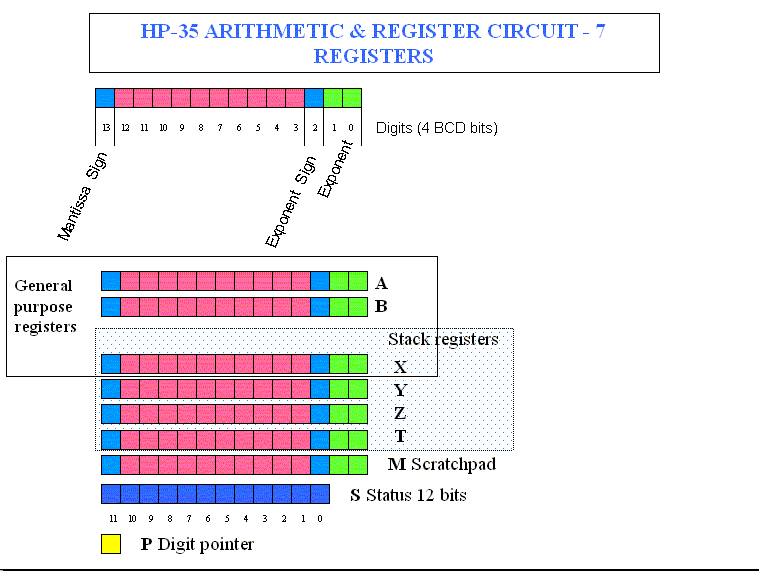Technically, in the ROM mnemonics, they were named differently (here I use and adapt David G. Hicks’s work):

- A, B: General purpose registers,
- C:  Display,
- D, E, F: operational stack levels Y, Z, and T,
- M: scratchpad register (transfers to and from the C register),
- P:  4 bit "pointer" register used for register field addressing (offset into the registers),
- S0-S11: 12 bits of programmable status.

Operations with a field select option allow part of each register to be accessed.

 M Mantissa MS Both mantissa and Sign X Exponent XS Exponent Sign S Mantissa Sign P Pointer W Word (the 14 digits of the specified register) WP Word up to the nibble pointed to by P register (if P=3, WP would refer to nibbles 0, 1, 2, and 3).

The sign nibbles are set to 0 if positive and 9 if negative.

The HP35 used 8 bit instruction addresses plus ROM select instructions to activate the appropriate 256 word ROM. Portions of registers to act on are indicated by enclosing the field select code in brackets []. Each instruction occupied 10 bits (256 x 10 = 2560 bits).

Here again to present the instruction set, see David G. Hicks for more details. I’ll focus on examples taken in the object code listing of the HP35 ROM.

 field field select ( M, MS,X, XS, S, P W, WP.) register general purpose register (A,B, C) label a label bit a value of 0 or 1 digit a value between 0 and 9 status a status register bit number (0 - 11) pointer a pointer value (0-13) rom a ROM number (max depends on model)

Clearing

 CLEAR REGISTERS Clear (zero) all 56 bit registers 0 -> register[field] Set the selected field of a register to zero. CLEAR STATUS Clear (zero) all 12 status bits

This is the CLR key routine and the PWO boot:

89   L00067:  111.1.1...        l00067:                clear registers
90   L00070:  .1.111...          1  -> L00134        jsb of12
132   L00134:  ..11..111.       of12:                   0 -> c[w]
133   L00135:  ....11.1..        of13:                  clear status

Register copy to register

 A -> B[field] B -> C[field] C -> A[field] M -> C C -> M Copy the selected field of the origin register to the field of the destination register

e.g.

The entry point for e^x ln log or x^y

02010: .11...111.                    c -> a[w]
A=04400000000000  B=02099999999999  C=04400000000000
D=00000000000000  M=00000000000000  P=c  S=0.2...6789.b

Register C (display) that contains the ln(x) argument, number 0.44 is saved in register A field W (entire word)

A few steps further:

02013: 1.111.111.                  0 -> a[w]
A=00000000000000  B=02099999999999  C=04400000000000
D=00000000000000  M=00000000000000  P=c  S=0.2...6789.b

The simulator gives the instruction in object and symbolic and the result of execution in A, B, C, D, M registers and also in the Pointer P and Status Bits (bits 0, 2, 6, 7, 8 9 are SET while others are reset).

Another example using field and pointer addressing (before and after execution):

A=01120000000000  B=00560000000000  C=04400000000000
D=00000000000000  M=00000000000000  P=c  S=0.2...6789.b

02024: 11.11...1.                    a - 1 -> a[p]
A=00120000000000  B=00560000000000  C=04400000000000
D=00000000000000  M=00000000000000  P=c  S=0.2...6789.b

Register A is decremented at the field pointed to by P (12) and the result is stored at he same address.

Register Exchange

 A EXCHANGE B[field] A EXCHANGE C[field] C EXCHANGE B[field] C EXCHANGE M Exchange fields of the two registers. C<>M always on full word.

e.g.

02331: 11...11111 -> 02307                 then go to mpy28
A=01481604541800  B=00000000000900  C=09900000000000
D=00000000000000  M=00000000000000  P=c  S=0.2...67.9.b

02332: 111.1..11.                 a exchange c[m]
A=09900000000800  B=00000000000900  C=01481604541000
D=00000000000000  M=00000000000000  P=c  S=0.2...67.9.b

Register A mantissa is exchanged with C counterpart

 Register Arithmetic A + B -> A[field] A + C -> A[field] A + C -> C[field] C + C -> C[field] A – B -> A[field] A – C -> C[field] A – C -> A[field] Perform arithmetic on field A + 1 -> A[field] Increment field C + 1 -> C[field] A – 1 -> A[field] Decrement field C – 1 -> C[field] -C -> C[field] Negation of C (10s complement) -C -1 -> C[field] Negation of C and decrement (9s complement)

e.g. eca22 routine

02023: 1..1.11..1 -> 02226     jsb eca22

A=22232000000000  B=22232000000000  C=11144000000000
D=00000000000000  M=00000000000000  P=c  S=0.2...6789.b

02226: 11.111111.          eca22:         a - 1 -> a[s]

A=12232000000000  B=22232000000000  C=11144000000000
D=00000000000000  M=00000000000000  P=c  S=0.2...6789.b

Mantissa sign of register A is decremented.

Register Shift

 SHIFT RIGHT register[field] Shift selected portion of register to the right SHIFT LEFT A[field] Shift selected portion of A to the left

02227: 1..1.1.111 -> 02225    if no carry go to eca21

A=12232000000000  B=22232000000000  C=11144000000000
D=00000000000000  M=00000000000000  P=c  S=0.2...6789.b

02225: 1.11.1..1.          eca21:            shift right a[wp]
A=10223200000000  B=22232000000000  C=11144000000000
D=00000000000000  M=00000000000000  P=c  S=0.2...6789.b

Routine eca21

Part of the A register (from 0 to 11 – P=12) is shifted right.

Register branching

 IF B[field] = 0 IF C[field] = 0 IF A >= C[field] IF A >= B[field] IF A[field] >= 1 IF B[field] >= 1 Test for selected part of  register (THEN GO TO) THEN GO TO label Go to label if true GO TO label Unconditional go to IF NO CARRY GO TO label Go to label if carry bit is not set

02226: 11.111111.          eca22:         a - 1 -> a[s]

A=00223200000000  B=22232000000000  C=11144000000000
D=00000000000000  M=00000000000000  P=c  S=0.2...6789.b

02227: 1..1.1.111 -> 02225    if no carry go to eca21

A=00223200000000  B=22232000000000  C=11144000000000
D=00000000000000  M=00000000000000  P=c  S=0.2...6789.b

02225: 1.11.1..1.          eca21:            shift right a[wp]

A=00022320000000  B=22232000000000  C=11144000000000
D=00000000000000  M=00000000000000  P=c  S=0.2...6789.b

In eca22, if operation decrementing sign of A does not generate a CARRY then GOTO eca21.

Status testing

 bit -> status Set status bit (eg: 1 -> S10) IF status = 0 Test if status bit equal to 0 IF status # 1 Test if status bit not equal to 1 )

At the very start of the ln routine, a test to status bit S8 is done ; if S8=0 then branch to exp(x) routine. It is in fact the entry point for e^x, ln, log or x^y.

02011: 1....1.1..          if s8 = 0

A=04400000000000  B=02099999999999  C=04400000000000
D=00000000000000  M=00000000000000  P=c  S=0.2...6789.b

"pointer" register setting

 pointer -> P Set P register to pointer (pointer nth nibble for P field select or through pointer for WP) P + 1 -> P P -1 -> P Increment and decrement pointer P IF P # pointer Test if P not equal to pointer (follow with THEN)

In prologue to ln(x), set P to 12.

00137: 11....11..          of14:    12 -> p

A=00000000000000  B=00000000000000  C=00000000000000
D=00000000000000  M=00000000000000  P=c  S=............

Stack operations

 C -> STACK Push C onto the stack (E->F, D->E, C->D) always whole word STACK -> A Pop stack into A (D->A, E->D, F->E) always whole word ROTATE DOWN Move D to C, E to D, F to E and the original C to F (just like pressing the Roll Down key.)

Subroutine call

02023: 1..1.11..1 -> 02226     jsb eca22

A=00560000000000  B=00560000000000  C=04400000000000
D=00000000000000  M=00000000000000  P=c  S=0.2...6789.b

02226: 11.111111.          eca22:         a - 1 -> a[s]

A=90560000000000  B=00560000000000  C=04400000000000
D=00000000000000  M=00000000000000  P=c  S=0.2...6789.b

Miscellaneous

 LOAD CONSTANT digit digit -> C at the Pth nibble. P is then decremented so multiple digits can be loaded easily. SELECT ROM rom Select ROM #rom for addressing. KEYS -> ROM ADDRESS Jump to the offset of the current keycode in the currently selected ROM. DISPLAY OFF Turn the display off DISPLAY TOGGLE Toggle the LED display on/off. NO OPERATION Place holder doing nothing.

Here we load the constant ln(10) = 0230258509

02366: ..11..111.          lnc10: 0 -> c[w]

A=00820980552045  B=44000000000000  C=00000000000000
D=00000000000000  M=00000000000000  P=b  S=0.2...67.9.b

02367: 11....11..                     12 -> p

A=00820980552045  B=44000000000000  C=00000000000000
D=00000000000000  M=00000000000000  P=c  S=0.2...67.9.b

A=00820980552045  B=44000000000000  C=02000000000000
D=00000000000000  M=00000000000000  P=b  S=0.2...67.9.b

A=00820980552045  B=44000000000000  C=02300000000000
D=00000000000000  M=00000000000000  P=a  S=0.2...67.9.b

A=00820980552045  B=44000000000000  C=02300000000000
D=00000000000000  M=00000000000000  P=9  S=0.2...67.9.b

Here we have pressed the LN key so we go offset 00003 (see table below).

00330: ..11.1....          keys -> rom address

A=44000000000000  B=02099999999999  C=04400000000000
D=00000000000000  M=00000000000000  P=c  S=0.....678..b

00003: .....1.111 -> 00005      go to l00005

A=44000000000000  B=02099999999999  C=04400000000000
D=00000000000000  M=00000000000000  P=c  S=0.....678..b

 "CLR", 0 "e^x", 2 "ln", 3 "log", 4 "x^y", 6 "RCL", 10 "STO", 12 "Rdwn", 13 "x<>y", 14 "1/x", 16 "6", 22 "5", 23 "4", 24 "+", 26 "3", 32 "2", 33 "1", 34 "x", 36 "Pi", 42 ".", 43 "0", 44 "/", 46 "tan", 50 "cos", 52 "sin", 53 "arc", 54 "sqrt(x) 56 "9", 62 "8", 63 "7", 64 "-", 66 "CLX", 70 "EEX", 72 "CHS", 73 "ENTER^ 76